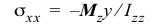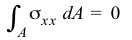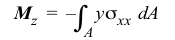# 3.3 – THEORY OF SYMMETRIC BENDING OF BEAMS

 In the questions below, select the correct answer. NameEmail1) If you know the bending normal strain at one point other than the centroid, then the bending normal strain can be found at any point on the cross section. True False2) If you the maximum bending normal stress on a cross section, then the bending normal stress at any point on the cross section can be found for a linear-homogenous material. True False3) A rectangular beam with a 2-in. × 4-in. cross section should be used with the 2-in. side parallel to the bending (transverse) forces. True False4) The best place to drill a hole in a beam is through the centroid. True False5) In the formulay is measured from the bottom of the beam. True False6) The formulacan be used to find the normal stress on a cross section of a tapered beam. True False7) The equationsandcannot be used for nonlinear materials. True False8) The equationcan be used for nonhomogeneous cross sections. True False9) The internal shear force jumps by the value of the applied transverse force as one crosses it from left to right. True False10) The internal bending moment jumps by the value of the applied concentrated moment as one crosses it from left to right. True FalseDo you have any comments or suggestions? Please email them to author@madhuvable.org